# How to utilise Pandas dataframe and series for data wrangling?

• Last Updated : 23 May, 2022

In this article, we are going to see how to utilize Pandas DataFrame and series for data wrangling.

The process of cleansing and integrating dirty and complicated data sets for easy access and analysis is known as data wrangling. As the amount of data raises continually and expands, it is becoming more important to organize vast amounts of data for analysis. Data wrangling comprises activities such as data sorting, data filtering, data reduction, data access, and data processing. Data wrangling is one of the most important tasks in data science and data analysis. Let’s see how to utilize Pandas DataFrame and series for data wrangling.

## Utilize series for data wrangling

### Creating Series

pd.Series() method is used to create a pandas Series. In this, a list is given as an argument and we use the index parameter to set the index of the Series. The index helps us to retrieve data based on conditions.

## Python3

 `# importing packages` `import` `pandas as pd`   `# creating a series` `population_data ``=` `pd.Series([``1440297825``, ``1382345085``,` `                             ``331341050``,` `                             ``274021604``, ``212821986``],` `                            ``index``=``[``'China'``, ``'India'``, ` `                                   ``'United States'``,` `                                   ``'Indonesia'``, ``'Brazil'``])`   `print``(population_data)`

Output: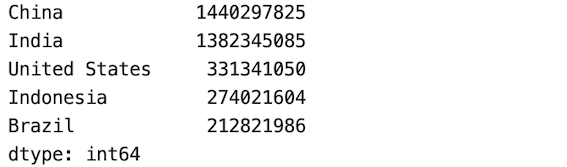### Filtering data- Retrieving insights based on conditions from the data

From the previous data, we retrieve data on two conditions, one is the population of India and another is countries that have a population of more than a billion.

## Python3

 `print``('population of india ``is` `: \` `' + str(population_data['``India']))`   `print``(``'population greater than a billion :'``)` `print``(population_data[population_data > ``1000000000``])`

Output: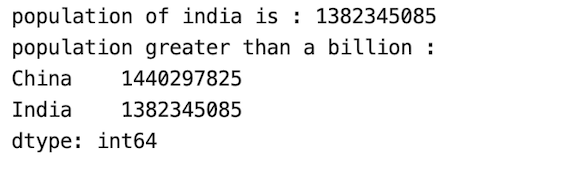We can also use dictionaries to create Series in python. In this, we have to pass a Dictionary as an argument in the pd.Series() method.

## Python3

 `population_data ``=` `pd.Series({``'China'``: ``1440297825``,` `                             ``'India'``: ``1382345085``, ` `                             ``'United States'``: ``331341050``, ` `                             ``'Indonesia'``: ``274021604``,` `                             ``'Brazil'``: ``212821986``})` `print``(population_data)`

Output:### Changing  indices by altering the index of series

In pd.Series the index can be manipulated or altered by specifying a new index series.

## Python3

 `population_data.index ``=` `[``'CHINA'``,``'INDIA'``,` `                         ``'US'``,``'INDONESIA'``,` `                         ``'BRAZIL'``]` `print``(population_data)`

Output: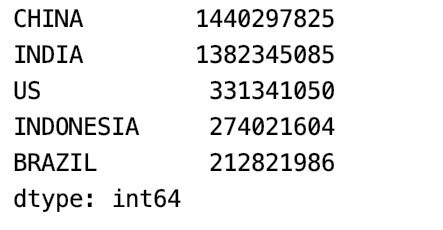## Utilize Pandas Dataframe for data wrangling

### Creating Dataframe using CSV

In this example, we will use a CSV file to print top n (5 by default) rows of a DataFrame or series using the Pandas.head() method.

## Python3

 `# importing packages` `import` `pandas as pd`   `# loading csv data` `population_data ``=` `pd.read_csv(``'employees.csv'``)`   `# setting the index of the dataframe` `population_data``=``population_data.set_index(``'First Name'``)`   `# head of the dataframe` `population_data.head()`

Output: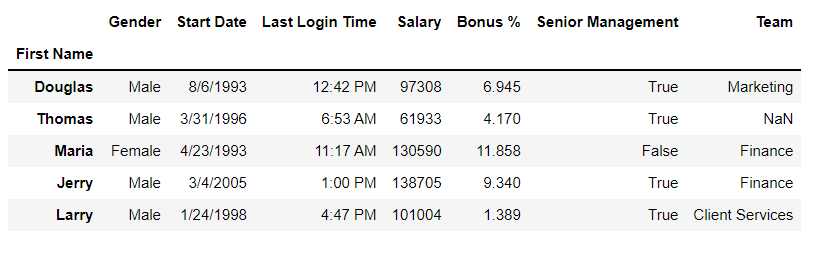### Describing DataFrame

pd.Describe() method is used to get the summary statistics of the Dataframe.

## Python3

 `# importing packages` `import` `pandas as pd`   `# loading csv data` `population_data ``=` `pd.read_csv(``'employees.csv'``)`   `population_data.describe()`

Output: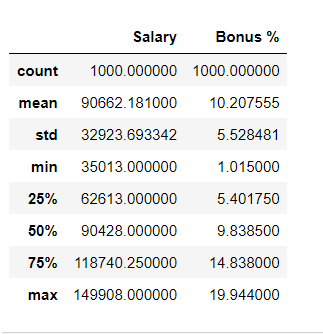### Setting and Resetting the index of the Dataframe

pd.set_index is used for setting and resetting the index of the Dataframe. Whereas, pd.reset_index() reverts the Dataframe back to the normal state. Here, the name of the column is given as an argument.

Example 1: Resetting the index of the Dataframe in Start Date columns

## Python3

 `# importing packages` `import` `pandas as pd`   `# creating a pandas Dataframe` `population_data ``=` `pd.read_csv(``'employees.csv'``)`   `# setting the index of the dataframe` `population_data ``=` `population_data.set_index(``'Start Date'``)` `pd.DataFrame(population_data)`

Output: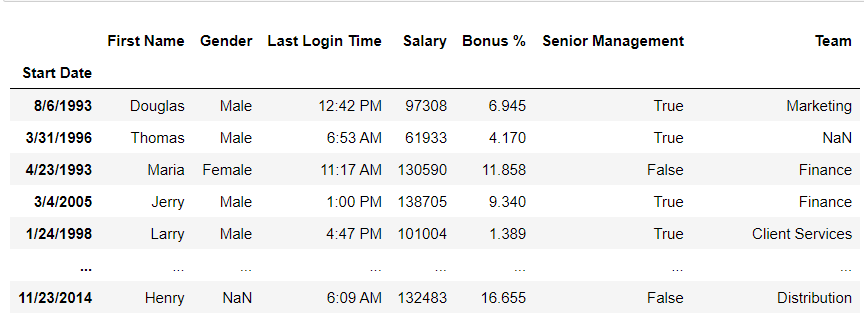Example 2: Resetting the index of the Dataframe in First Name columns

## Python3

 `# importing packages` `import` `pandas as pd`   `# creating a pandas Dataframe` `population_data ``=` `pd.read_csv(``'employees.csv'``)`     `# resetting the index of the dataframe` `population_data ``=` `population_data.reset_index()`   `population_data ``=` `population_data.set_index(``'First Name'``)`   `pd.DataFrame(population_data)`

Output: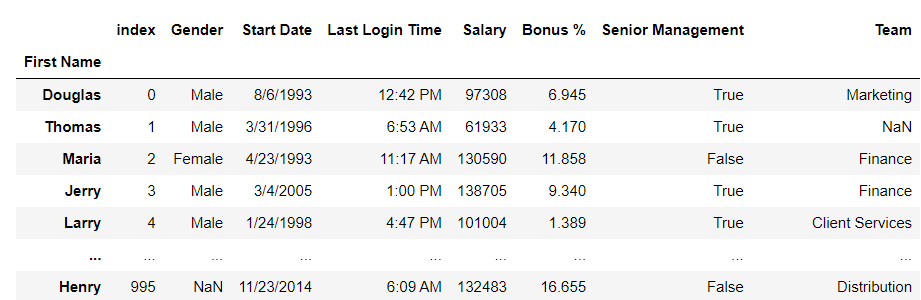### Deleting a column from the DataFrames

The column ‘Salary’ is deleted from the DataFrames from our CSV file.

## Python3

 `# importing packages` `import` `pandas as pd`   `# loading csv data` `population_data ``=` `pd.read_csv(``'employees.csv'``)`     `# deleting column` `del` `population_data[``'Salary'``]` `                    `  `pd.DataFrame(population_data.head())`

Output: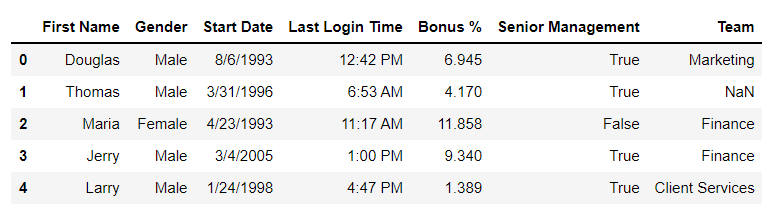### Reshaping dataframe

df.Transpose() function is used to find the transpose of the given DataFrame.

## Python3

 `# importing packages` `import` `pandas as pd`   `# loading csv data` `population_data ``=` `pd.read_csv(``'employees.csv'``)`   `# setting the index of the dataframe` `population_data``=``population_data.set_index(``'First Name'``)`   `# displaying a transpose of the dataframe` `pd.DataFrame(population_data.transpose().head())`

Output: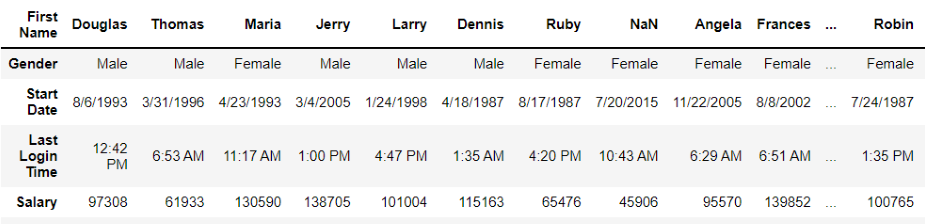### Sorting the Dataframe

df.sort_values() function is used to sort data. In this, the column name is passed as a parameter.

## Python3

 `# importing packages` `import` `pandas as pd`   `# loading csv data` `population_data ``=` `pd.read_csv(``'employees.csv'``)`   `# setting the index of the dataframe` `population_data``=``population_data.set_index(``'First Name'``)`   `# sorting the Dataframe based on Density of population per km column` `sorted_dataframe ``=` `population_data.sort_values(``'Salary'``, ascending``=``False``)` `pd.DataFrame(sorted_dataframe)`

Output: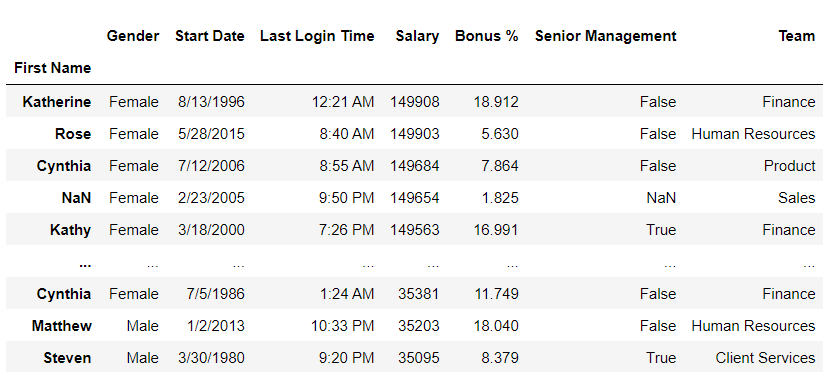### Dealing with missing values

Missing or null values can be checked with the Pandas df.null() method.

## Python3

 `# importing packages` `import` `pandas as pd`   `# loading csv data` `data ``=` `pd.read_csv(``'employees.csv'``)`   `# checking for null values` `data.isnull().``sum``()`

Output: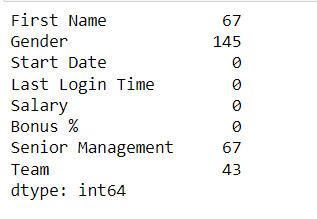### Dropping Rows

We can filter rows that have null values by using df.dropna() method.

## Python3

 `# importing packages` `import` `pandas as pd`   `# loading csv data` `data ``=` `pd.read_csv(``'employees.csv'``)`   `# dropping NA values` `data ``=` `data.dropna(axis``=``0``, how``=``'any'``)`   `# checking for null values` `data.isnull().``sum``()`

Output: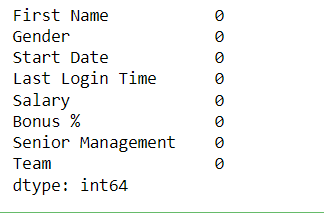### Grouping Data

In Data Analysis, the Grouping of data sets is a common requirement when the outcome must be expressed in terms of many groups. Panadas provides us with a built-in mechanism for grouping data into several categories. The pandas df.groupby() technique is used for grouping data.

In the below code, We will create a DataFrames of students and their grades. In this groupby() method is used to group students according to their grades with their names.

## Python3

 `# importing pandas as pd` `import` `pandas as pd`   `# Creating the dataframe` `df ``=` `pd.read_csv(``"employees.csv"``)`   `# First grouping based on "Team"` `# Within each team we are grouping based on "Position"` `data ``=` `df.groupby([``'First Name'``, ``'Gender'``])`   `# Print the first value in each group` `data.first()`

Output: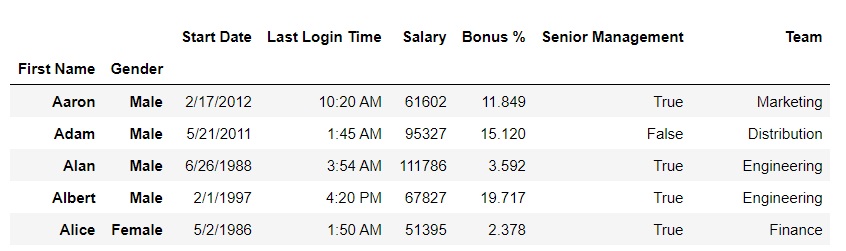### Merging Dataframe

Pandas df.merge() method is used to merge two DataFrames. There are different ways of merging DataFrames like, outer join, inner join, left join, right join, etc.

## Python3

 `import` `pandas as pd`   `# reading two csv files` `data ``=` `pd.read_csv(``'employees.csv'``)`   `# creating two dataframe ` `head_data ``=` `data.head()` `tail_data ``=` `data.tail()`   `# get top 5 rows` `print``(``"Head Data :"``)` `display(head_data)`   `# get last 5 rows` `print``(``"Tail Data :"``)` `display(tail_data)`   `# merge dataframke` `merge_data ``=` `pd.merge(head_data, tail_data, how``=``'outer'` `)`   `print``(``"After merging: "``)` `display(merge_data)`

Output: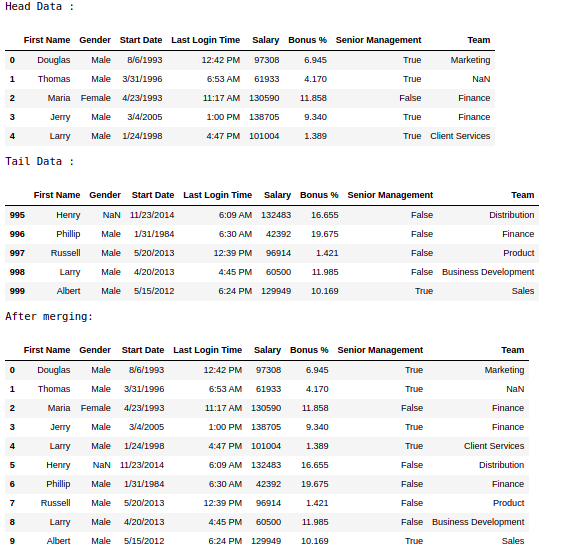### Concatenating Data

The Concat function is used to conduct concatenation operations along an axis. Let’s create two DataFrames and concatenate them.

## Python3

 `import` `pandas as pd`   `# reading two csv files` `data1 ``=` `pd.read_csv(``'employees.csv'``)` `data2 ``=` `pd.read_csv(``'borrower.csv'``)`   `# concatenating the dataframes` `pd.DataFrame(pd.concat([data1,data2]))`

Output: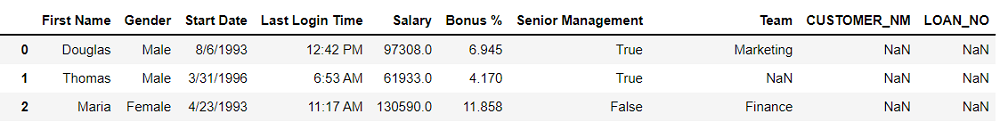My Personal Notes arrow_drop_up
Recommended Articles
Page :